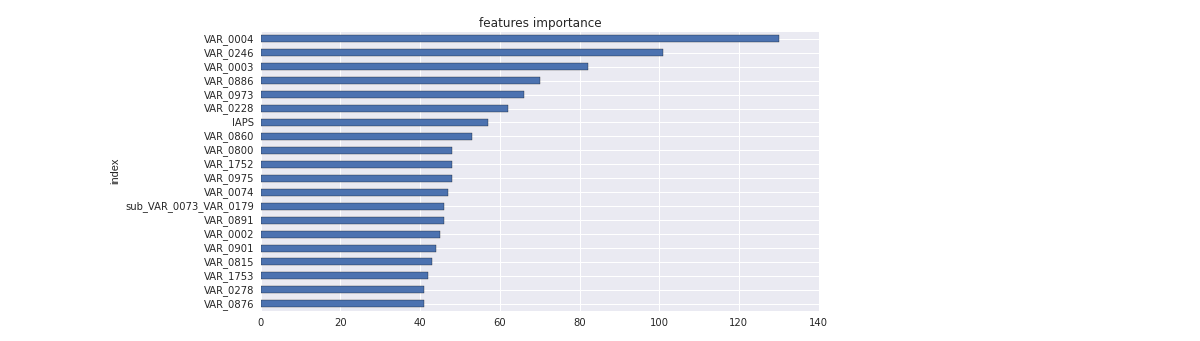howto

Advanced XGBoost tuning in Python

Applies to DSS 3.0 and above | August 22, 2016

XGBoost is an advanced gradient boosting tree library. It is integrated into Dataiku DSS visual machine learning, meaning that you can train XGBoost models without writing any code.

In this How-To, we are going to cover some advanced optimization techniques that can help you go even further with your XGBoost models, by using custom Python code.

We assume that you are already familiar with how to train a model using Python code (for example with scikit-learn).

## Parameters

Most of these parameters are directly available when you create a XGBoost model using the visual machine learning component of DSS: you don't actually need to code for this part.

XGBoost has a large number of advanced parameters, which can all affect the quality and speed of your model.

max_depth : int
Maximum tree depth for base learners.
learning_rate : float
Boosting learning rate (XGBoost's "eta")
n_estimators : int
Number of boosted trees to fit.
silent : boolean
Whether to print messages while running boosting.
objective : string
Specify the learning task and the corresponding learning objective.
Number of parallel threads used to run XGBoost.
gamma : float
Minimum loss reduction required to make a further partition
on a leaf node of the tree.
min_child_weight : int
Minimum sum of instance weight(hessian) needed in a child.
max_delta_step : int
Maximum delta step we allow each tree's weight estimation to be.
subsample : float
Subsample ratio of the training instance.
colsample_bytree : float
Subsample ratio of columns when constructing each tree.
base_score:
The initial prediction score of all instances, global bias.
seed : int
Random number seed.
missing : float, optional
Value in the data which needs to be present as a missing value.
If None, defaults to np.nan.

You have 2 ways to control overfitting in XGBoost:

• Control the model complexity with max_depth, min_child_weight and gamma.
• Add randomness to make training robust to noise with subsample and colsample_bytree.

Using a Sparse matrix

XGBoost can take a sparse matrix as input. This allows you to convert categorical variables with high cardinality into a dummy matrix, then build a model without getting an out of memory error!

For this we use a Python function:

This returns a sparse matrix of 3 columns, one by value of VAR_0001:

You can concatenate this matrix with another dummy matrix with the SciPy hstack function:

Early stopping.

When creating a XGBoost model using the visual machine learning component of Dataiku DSS, it automatically uses early stopping (you don't actually need to code to benefit from this).

A really cool feature of XGBoost is early stopping. As you train more and more trees, you will overfit your training dataset. Early stopping enables you to specify a validation dataset and the number of iterations after which the algorithm should stop if the score on your validation dataset didn’t increase.

To use it, you can specify in the fit method of the classifier an evaluation set, an evaluation method and the early stopping round number:

Here, we explicitly set n_estimators to a very large number. In your job log you’ll see the score increasing on the dataset you put in the eval_set list:

Note that you can define your own evaluation metric instead.

Viewing features importance.

You can get the features importance easily in clf.booster().get_fscore() where clf is your trained classifier.

For example, we can use this in a Jupyter notebook:Using Hyperopt for grid searching

Fine-tuning your XGBoost can be done by exploring the space of parameters possibilities. For this task, you can use the hyperopt package. Hyperopt is a Python library for optimizing over awkward search spaces with real-valued, discrete, and conditional dimensions.

Here an example python recipe to use it:

After loading your training and validation datasets, we define our objective function.

This function trains a model, evaluates it and returns the error on the validation set. We define the space we want to explore: here, we want to try values from 5 to 30 for max_depth, from 1 to 10 for min_child_weight and from 0.8 to 1 for subsample.

Hyperopt will minimize this error in a maximum of 100 experiments.

See the Hyperopt documentation for more details.